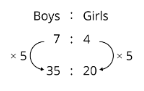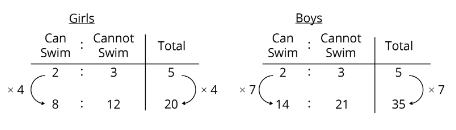Study P5 Mathematics Ratio Strategy 1: Repeated Identity - Geniebook# Ratio Strategy: Repeated Identity

In this article, we are going to learn about how to solve repeated identity questions.  To solve questions on repeated identity,

Step 1:

Write down the two sets of ratios.

Step 2:

Identify the item that is repeated.

Step 3:

Make the units for the repeated item the same in the 2 sets of ratio by finding the common multiple.

Step 4:

Read the question carefully and assign a value to the units if necessary.

Step 5:

Solve for 1 unit if necessary and answer the question.

Question 1:

The ratio of the number of red pens to the number of blue pens in a box is 3 : 4. The ratio of the number of red pens to the number of black pens in the same box is 2 : 3. What is the ratio of the number of red pens to the number of blue pens to the number of black pens?

Solution:

The number of red pens is the repeated identity.

We make the units representing the red pens the same by finding the common multiple of 3 and 2.6 : 8 : 9

Question 2:

Mrs Koh bought some apples, oranges, and pears. The ratio of the number of apples to the number of oranges was 3 : 2. The ratio of the number of pears to the number of apples was 5 : 2. Mrs Koh bought 18 apples. How many pears did she buy?

Solution:

The number of apples is the repeated identity.

We make the units representing the apples the same by finding the common multiple of 3 and 2.\begin{align*} \because \end{align*}    6 units = 18

\begin{align*} \therefore \end{align*}    1 unit   = 18 ÷ 6

= 3

Number of pears = 15 units

= 15 × 3

= 45

45 pears

### Question 3:

The ratio of the amount of money Aini has to the amount of money Siti has is 3 : 1. The ratio of the amount of money Aini has to the amount of money Winnie has is 1 : 2. If the three girls have a total of 60, how much money does Winnie have? Solution: Aini is the repeated identity. We make the units representing Aini the same by finding the common multiple of 3 and 1.Total number of units the three girls have = 3 units + 1 unit + 6 units = 10 units \begin{align*} \because \end{align*} 10 units =60

\begin{align*} \therefore \end{align*}    1 unit     = $60 ÷ 10 =$6

Amount of money Winnie has = 6 units

= 6 × $6 =$36

\$36

Question 4:

The diagram shows four points A, B, C, and D in a straight line. AB is \begin{align*} \frac { 5 } { 9 } \end{align*} as long as AC and CD is \begin{align*} \frac { 2 } { 5 } \end{align*} as long as BD.A) What is the ratio of the length of AB to the length of BC to the length of CD?

Solution:

BC is the repeated identity.

We make the units representing BC the same by finding the common multiple of 4 and 3.15 : 12 : 8

B) Given that CD = 48 cm, what is the length of AD?

Solution:

\begin{align*} \because \end{align*}    8 units = 48 cm

\begin{align*} \therefore \end{align*}    1 unit   = 48 cm ÷ 8

= 6 cm

Length of AD = 15 units + 12 units + 8 units

= 35 units

= 35 × 6 cm

= 210 cm

210 cm

Question 5:

\begin{align*} \frac { 9 } { 20 } \end{align*} of the passengers on a plane are adults and the rest are children. The ratio of the number of boys to the number of girls is 2 : 3.

A) What is the ratio of the number of adults to the number of boys to the number of girls on the plane?

Solution:

The number of children is the repeated identity.

We make the units representing the number of children the same by finding the common multiple of 11 and 5.45 : 22 : 33

B) There are 36 more adults than girls. How many passengers are there on the plane

Solution:

Difference in number of units between the number of adults and the number of girls

= 45 units – 33 units

= 12 units

\begin{align*} \because \end{align*}     12 units = 36

\begin{align*} \therefore \end{align*}     1 unit     = 36 ÷ 12

= 3

Total number of passengers = 45 units + 22 units + 33 units

= 100 units

= 100 × 3

= 300

300 passengers

Question 5:

\begin{align*} \frac { 7 } { 11 } \end{align*} of the pupils in a school are boys. \begin{align*} \frac { 2 } { 5 } \end{align*} of the girls can swim. The ratio of the number of boys who can swim to the number of boys who cannot swim is 2 : 3.

A) What is the ratio of the number of pupils who can swim to the number of pupils who cannot swim in the school?

Solution:The total number of girls is repeated.

We make the units representing the girls the same by finding the common multiple of 5 and 20.

The total number of boys is also repeated.

We make the units representing the boys the same by finding the common multiple of 5 and 35.Number of units of pupils who can swim = 8 units + 14 units

= 22 units

Number of units of pupils who cannot swim = 12 units + 21 units

= 33 units2 : 3

B) 330 pupils in the school cannot swim. How many girls are there in the school?

Solution:

\begin{align*} \because \end{align*}    33 units = 330

\begin{align*} \therefore \end{align*}    1 unit     = 330 ÷ 33

= 10

Number of girls = 20 units

= 20 × 10

= 200

200 girls

Continue Learning
Volume Of A Liquid Decimals - Operations & Conversions
Ratio: Introduction Average - Formula
Percentage, Fractions And Decimals Whole Numbers
Strategy - Equal Stage Angle Properties
Table Rates Whole Number Strategy: Gap & Difference
Fractions - Addition & Subtraction Ratio Strategy: Repeated IdentityPrimaryPrimary 1Primary 2Primary 3Primary 4Primary 5EnglishMaths
Volume Of A Liquid
Decimals - Operations & Conversions
Ratio: Introduction
Average - Formula
Percentage, Fractions And Decimals
Whole Numbers
Strategy - Equal Stage
Angle Properties
Table Rates
Whole Number Strategy: Gap & Difference
Ratio Strategy: Repeated IdentitySciencePrimary 6SecondarySecondary 1Secondary 2Secondary 3Secondary 4